## Cross-Correlation Theorem

Let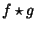denote the Cross-Correlation of functionsand. Then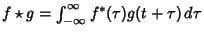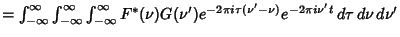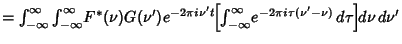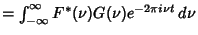(1)

wheredenotes the Fourier Transform and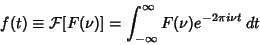(2)(3)

Applying a Fourier Transform on each side gives the cross-correlation theorem,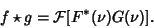(4)

If, then the cross-correlation theorem reduces to the Wiener-Khintchine Theorem.

See also Fourier Transform, Wiener-Khintchine Theorem

© 1996-9 Eric W. Weisstein
1999-05-25